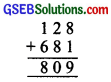# GSEB Solutions Class 8 Maths Chapter 16 Playing with Numbers Ex 16.1

Gujarat Board GSEB Textbook Solutions Class 8 Maths Chapter 16 Playing with Numbers Ex 16.1 Questions and Answers.

## Gujarat Board Textbook Solutions Class 8 Maths Chapter 16 Playing with Numbers Ex 16.1

Question 1.
Find the values of the letters in each of the following and give reasons for the steps involved?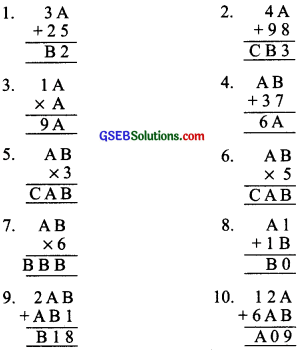Solution:
1. ∵ A + 5 =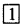2 or 22 or 32, etc.
∴ A = 12 – 5
= 7 or 22 – 5 = 17
Since A = 17 is not possible∴ A = 7
Since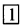+ 3 + 2 = 6
∴ B = 6
Thus, A = 7, B = 62. If A + 8 =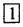3
Then A = 13 – 8 = 5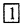+ 4 + 9 = B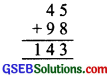or B =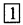4
Clearly C = 1
Thus A = 5, B = 4 and C = 1

3. ∵ A × A = 1
∴ A = 1, or A = 5
or A = 6If A = 1, then
∵ 11 ≠ 9A
∴ A = 1, or A = 5
75 ≠ 9A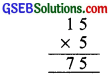∴ A = 5
If A = 6, then
∵ 96 = 9A
∴ A = 6 is the required values of A.4. We need B + 7 = A
∴ Possible value of B can be 5,
[∵ 5 + 7 = 12]
∵ 6 – 3 – 1 = 2
∴ A can be equal to 2
Now
∴ A = 2 and B = 5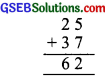5. We need B × 3 =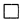B
Since 5 × 3 = 15
∴ Possible value of B can be 5.
Also 0 × 3 = 0, i.e; B = 0 can be another possible value.
∵ A × 3 = A + 0 = A
∴ Possible value of A = 5 or A = 0
∴ Since C ≠ 0
∴ Possible value of A = 5
30, B must be
Thus, B = 0.
∴ A = 5, B = 0 and C = 1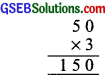6. B can be either 5 or 0.
∵ A × 5 = A
∴ B must be o
Again A can either be 5 0r 0.
∴ C ≠ 0 ∴ A ≠ 0
∴ A must be equal to 5
Thus, we have
Therefore, A = 5, B = 0, C = 2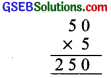7. Be can be 2, 4, 6 or 8
We need product 111 or 222, or 333 or 444
or 888 out of them 111 and 333 are rejected.
Possible products are 222, 444 or 888
To obtain
The possible value is B = 4∴ A can be either 2 or 7
A × 6 means 2 × 6 = 12 or 7 × 6 = 42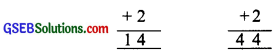∴ A × 6 = 7 × 6 is the accepted value
Now,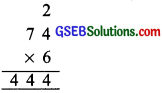Thus, A = 7 and B = 48. ∵ 10 – 1 = 9
∴ B = 9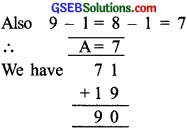Thus, A = 7 and B = 9

9. ∵ 8 – 1 = 7
∴ B = 7
∴ 7 + 4 = 11 ∴ A = 4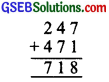Now, we have
Thus, A = 4 and B = 710. ∵ 10 – 2 = 8
∴ A = 8
Also, 9 – 8 = 1
∴ B = 1
Now, we have
Thus, A = 8 and B = 1